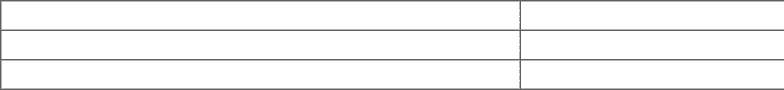Class Notes (1,100,000)
CA (620,000)
Queen's (10,000)
CISC (500)
Lecture 2

# CISC 235 Lecture Notes - Lecture 2: Random-Access Machine, Complex Instruction Set Computing, Time Complexity

Department
Computing
Course Code
CISC 235
Professor
Robin W Dawes
Lecture
2

This preview shows pages 1-2. to view the full 6 pages of the document.CISC 235 Lecture 2
What criterion should we use to choose an appropriate data
structure for an application?
How about "I already understand data structure A, and I don't
understand data structure B"? .... umm, no.
Perhaps "There is a built-in module for data structure A, but I
would have to code data structure B myself"? .... fail!
What could be left? What could be right?
Why, computational complexity, of course! We will prefer
structure A to structure B if A has a lower order of complexity for
the operations we need in our particular application.
Before we discuss computational complexity, we need to agree on
the type of computer we are dealing with. In particular, we need to
clarify which operations can be completed in constant time.
The R.A.M. model of computation
We will use the Random Access Machine model, which can be
summarized as follows:
- sequential operations only (i.e. no parallel computation)
- all fundamental operations:
- +, -, *, / and comparisons for integers and floating
point numbers
- comparisons on booleans
- comparisons and type conversions on characters
- execution control

Only pages 1-2 are available for preview. Some parts have been intentionally blurred.- accessing a memory address
- assigning a value to a variable
take constant time
- no hardware accelerators (such as caches or virtual
memory) are employed
It is important to note that this model implies an upper limit on the
number of digits in any number. This is true of virtually all
programming languages.
The RAM model does not assume constant time operations on
strings. A string is considered to be a data structure consisting of a
sequence of characters.
I'm assuming we are all familiar with "big O" complexity
classification (since it has been covered in other courses, most
notably CISC-203!):
Let f(n) and g(n) be non-negative valued functions on the set of
non-negative numbers. f(n) is in O(g(n)) if there are constants c
and n0 such that f(n) <= c*g(n) for all n >= n0
The significance of this is that as n gets large, the growth-rate of
f(n) is no greater than the growth rate of g(n). In other words, the
growth of g(n) is an upper bound on the growth of f(n).
There are several complexity classes that we encounter
frequently. Here is a table listing the most common ones.
Dominant Term
Big-O class
Description
c (a constant)
O(1)
constant time
###### You're Reading a Preview

Unlock to view full version